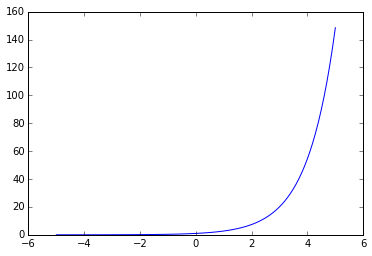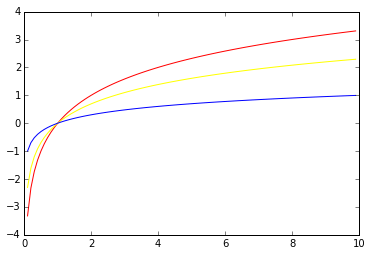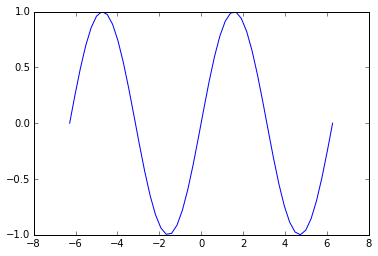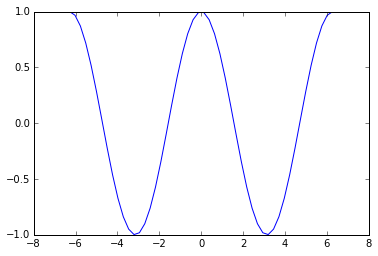# 函数

### 函数

#### 一些函数的例子：

1.多项式(polynomials)

$f(x)=x^3-5x^2+9$2.指数函数(Exponential Functions):

$exp(x)=e^x$$e^x = sum_{k = 0}^{infty}frac{x^k}{k!}$

3.对数函数(Logarithmic Functions):

$log_{e}(x)=ln(x)$

numpy为我们提供了以$2,e,10$为底的对数函数：4.三角函数(Trigonometric Functions):